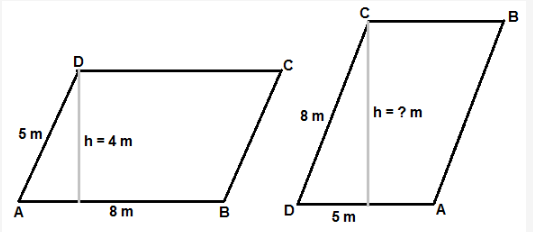# The adjacent side of parallelogram are 8m and 5m

The adjacent side of parallelogram are 8m and 5m. If the distance between the longer side is 4m, find the distance between the shorter side.left side of the figure shows a parallelogram of adjacent sides 8 m and 5 m drawn with 8 m side in horizontal.
It is given that distance between longer sides is 4 m. Hence area of parallelogram = base×height = 8×4 = 32 sq.m

Now right side of the figure shows, same parallelogram is drawn with shorter side as base.
since we know the area already as 32 sq.m, we can calculate the distance between shorter side as 5×h = 32 or h = 6.4 m